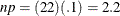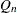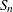### Example 4.11 Computing Robust Estimates

This example illustrates how you can use the UNIVARIATE procedure to compute robust estimates of location and scale. The following statements compute these estimates for the variable Systolic in the data set BPressure, which was introduced in Example 4.1:

title 'Robust Estimates for Blood Pressure Data';
ods select TrimmedMeans WinsorizedMeans RobustScale;
proc univariate data=BPressure trimmed=1 .1
winsorized=.1  robustscale;
var Systolic;
run;


The ODS SELECT statement restricts the output to the TrimmedMeans, WinsorizedMeans, and RobustScale tables; see the section ODS Table Names. The TRIMMED= option computes two trimmed means, the first after removing one observation and the second after removing 10% of the observations. If the value of TRIMMED= is greater than or equal to one, it is interpreted as the number of observations to be trimmed. The WINSORIZED= option computes a Winsorized mean that replaces three observations from the tails with the next closest observations. (Three observations are replaced because, and three is the smallest integer greater than 2.2.) The trimmed and Winsorized means for Systolic are displayed in Output 4.11.1.

Output 4.11.1: Computation of Trimmed and Winsorized Means

 Robust Estimates for Blood Pressure Data

The UNIVARIATE Procedure
Variable: Systolic

Trimmed Means
Percent
Trimmed
in Tail
Number
Trimmed
in Tail
Trimmed
Mean
Std Error
Trimmed
Mean
95% Confidence Limits DF t for H0:
Mu0=0.00
Pr > |t|
4.55 1 120.3500 2.573536 114.9635 125.7365 19 46.76446 <.0001
13.64 3 120.3125 2.395387 115.2069 125.4181 15 50.22675 <.0001

Winsorized Means
Percent
Winsorized
in Tail
Number
Winsorized
in Tail
Winsorized
Mean
Std Error
Winsorized
Mean
95% Confidence Limits DF t for H0:
Mu0=0.00
Pr > |t|
13.64 3 120.6364 2.417065 115.4845 125.7882 15 49.91027 <.0001

Output 4.11.1 shows the trimmed mean for Systolic is 120.35 after one observation has been trimmed, and 120.31 after 3 observations are trimmed. The Winsorized mean for Systolic is 120.64. For details on trimmed and Winsorized means, see the section Robust Estimators. The trimmed means can be compared with the means shown in Output 4.1.1 (from Example 4.1), which displays the mean for Systolic as 121.273.

The ROBUSTSCALE option requests a table, displayed in Output 4.11.2, which includes the interquartile range, Gini’s mean difference, the median absolute deviation about the median,, and.

Output 4.11.2 shows the robust estimates of scale for Systolic. For instance, the interquartile range is 13. The estimates ofrange from 9.54 to 13.32. See the section Robust Estimators.

A sample program for this example, uniex01.sas, is available in the SAS Sample Library for Base SAS software.

Output 4.11.2: Computation of Robust Estimates of Scale

Robust Measures of Scale
Measure Value Estimate
of Sigma
Interquartile Range 13.00000 9.63691
Gini's Mean Difference 15.03030 13.32026
MAD 6.50000 9.63690
Sn 9.54080 9.54080
Qn 13.33140 11.36786